Home > A2C > Chapter 2 > Lesson 2.2.2 > Problem2-142

2-142.
1. Multiply each pair of functions below to find an expression for f (x) · g(x). 2-142 HW eTool (Desmos). Homework Help ✎

1. f(x) = 2x, g(x) = (x + 3)

2. f(x) = (x + 3), g(x) = (x − 5)

3. f(x) = (2x + 1), g(x) = (x − 3)

4. f(x) = (x + 3), g(x) = (x + 3)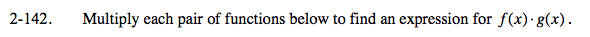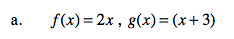Multiply the expressions.

(2x) (x + 3)

2x2 + 6x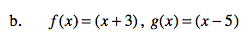Multiply using a generic rectangle.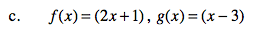See part (b).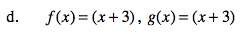See part (b).

Use the eTool below to help you multiply.
Click the link at right for the full version of the eTool: A2C 2-142 HW eTool